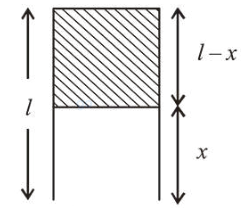# A parallel plate capacitor has plate of length ' l ', width ' w ' and separation of plates is ' d '.Question:

A parallel plate capacitor has plate of length ' $l$ ', width ' $w$ ' and separation of plates is ' $d$ '. It is connected to a battery of emf V. A dielectric slab of the same thickness ' $d$ ' and of dielectric constant $k=4$ is being inserved between the plates of the capacitor. At what length of the slab inside plates, will the energy stored in the capacitor be two times the initial energy stored?

1. (1) $2 l / 3$

2. (2) $l / 3$

3. (3) $l / 4$

4. (4) $l / 2$

Correct Option:

Solution:

(2) Capacitance before inserting the slab,

$C_{i}=\frac{\varepsilon_{0} A}{d}=\frac{\varepsilon_{0} l w}{d}$                          $(\because A=l w)$

Capacitance after inserting the dielectric slab,

$C_{f}=\frac{K \varepsilon_{0} A_{1}}{d}+\frac{\varepsilon_{0} A_{2}}{d}$

$=\frac{K \varepsilon_{0} W x}{d}+\frac{\varepsilon_{0} w(l-x)}{d}$According to question,

$2 \times$ Initial energy $=$ Final energy

$\Rightarrow\left(\frac{1}{2} C_{i} V^{2}\right) 2=\frac{1}{2} C_{f} V^{2}$

$\Rightarrow 2 C_{i}=C_{f}$

$\Rightarrow 2\left(\frac{\varepsilon_{0} w l}{d}\right)=\frac{\varepsilon_{0} K w x}{d}+\frac{\varepsilon_{0} w(l-x)}{d}$

$\Rightarrow 2 l=k x+(l-x) \Rightarrow x=\frac{l}{3}$